31 Parts of Lists
Part lets you pick out an element of a list.
 In:=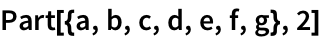Out=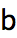[[...]] is an alternative notation.
Use [] to pick out element 2 from a list:
 In:=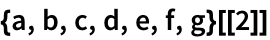Out=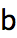In:=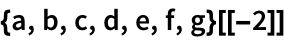Out=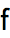You can ask for a list of parts by giving a list of part numbers.
Pick out parts 2, 4 and 5:
 In:=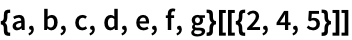Out=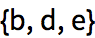;; lets you ask for a span or sequence of parts.
Pick out parts 2 through 5:
 In:=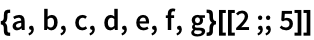Out=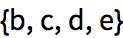In:=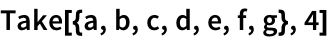Out=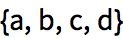Take the last 2 elements from a list:
 In:=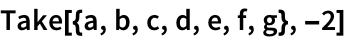Out=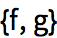Drop the last 2 elements from a list:
 In:=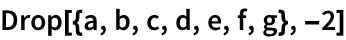Out=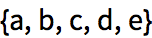In:=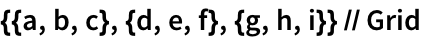Out=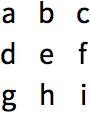Pick out the second sublist, corresponding to the second row, in the array:
 In:=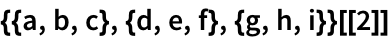Out=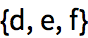This picks out element 1 on row 2:
 In:=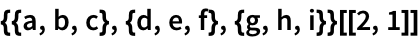Out=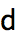Pick out the first column, by getting element 1 on all rows:
 In:=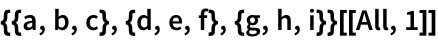Out=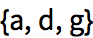The function Position finds the list of positions at which something appears.
 In:=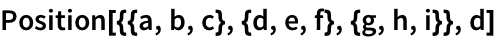Out=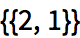This gives a list of all positions at which x appears:
 In:=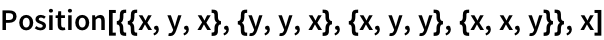Out=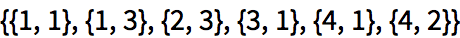The positions at which a occurs in a list of characters:
 In:=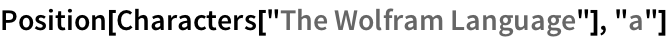Out=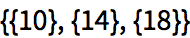In:=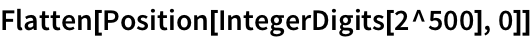Out=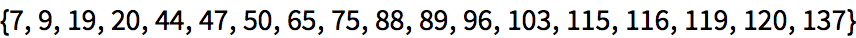The function ReplacePart lets you replace parts of a list:
Replace part 3 with x:
 In:=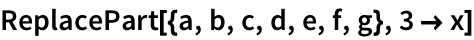Out=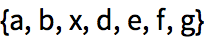Replace two parts:
 In:=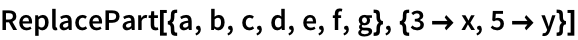Out=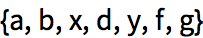In:=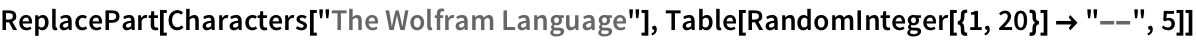Out=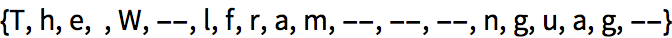Nothing just disappears:
 In:=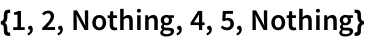Out=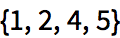Replace parts 1 and 3 with Nothing:
 In:=Out=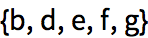Take 50 random words, dropping ones longer than 5 characters, and reversing others:
 In:=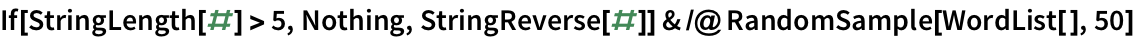Out=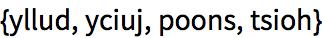Take takes a specified number of elements in a list based on their position. TakeLargest and TakeSmallest take elements based on their size.
Take the 5 largest elements from a list:
 In:=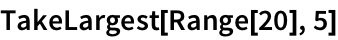Out=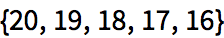TakeLargestBy and TakeSmallestBy take elements based on applying a function.
 In:=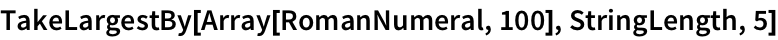Out=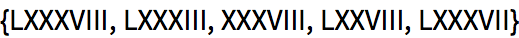Part[list,n] part n of a list list[[n]] short notation for part n of a list list[[{n1,n2,...}]] list of parts n1, n2, ... list[[n1;;n2]] span (sequence) of parts n1 through n2 list[[m,n]] element from row m, column n of an array list[[All,n]] all elements in column n Take[list,n] take the first n elements of a list TakeLargest[list,n] take the largest n elements of a list TakeSmallest[list,n] take the smallest n elements of a list TakeLargestBy[list,f,n] take elements largest by applying f TakeSmallestBy[list,f,n] take elements smallest by applying f Position[list,x] all positions of x in list ReplacePart[list,nx] replace part n of list with x Nothing a list element that is automatically removed
31.1Find the last 5 digits in 2^1000. »
Expected output:
 Out[]=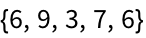Answer & check your solution
31.2Pick out letters 10 through 20 in the alphabet. »
Expected output:
 Out[]=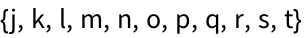Answer & check your solution
31.3Make a list of the letters at even-numbered positions in the alphabet. »
Expected output:
 Out[]=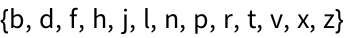Answer & check your solution
31.4Make a line plot of the second to last digit in the first 100 powers of 12. »
Expected output:
 Out[]=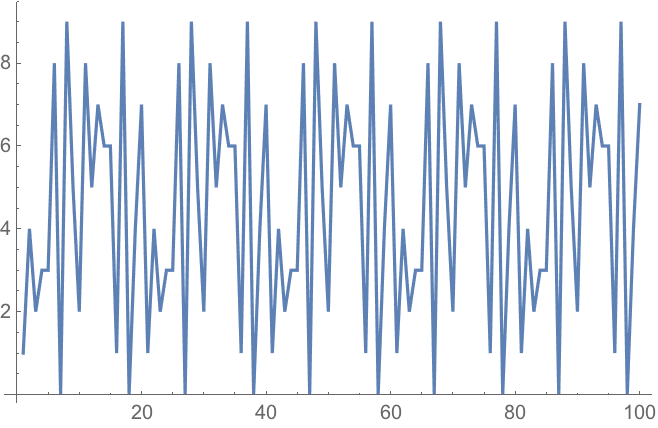Answer & check your solution
31.5Join lists of the first 20 squares and cubes, and get the 10 smallest elements of the combined list. »
Expected output:
 Out[]=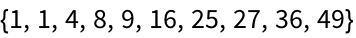Answer & check your solution
31.6Find the positions of the word software in the Wikipedia entry for computers»
Sample expected output:
 Out[]=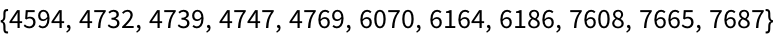Answer & check your solution
31.7Make a histogram of where the letter e occurs in the words in WordList[ ]»
Sample expected output:
 Out[]=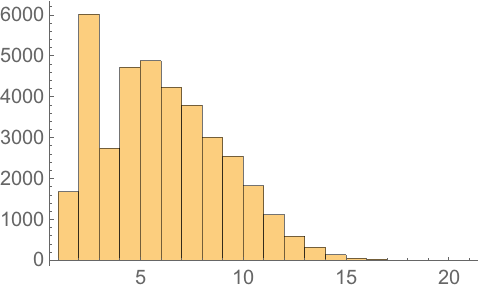Answer & check your solution
31.8Make a list of the first 100 cubes, with every one whose position is a square replaced by Red»
Expected output:
 Out[]=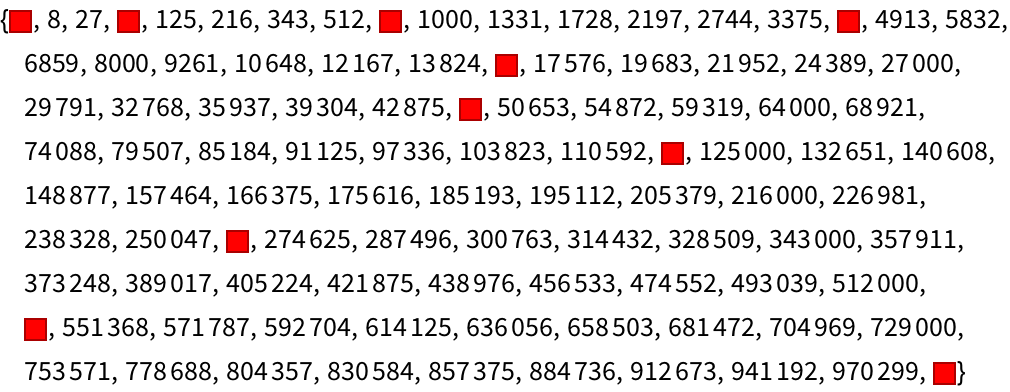Answer & check your solution
31.9Make a list of the first 100 primes, dropping ones whose first digit is less than 5. »
Expected output:
 Out[]=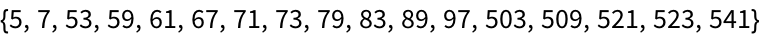Answer & check your solution
31.10Make a grid starting with Range, then at each of 9 steps randomly removing another element. »
Sample expected output:
 Out[]=Answer & check your solution
31.11Find the longest 10 words in WordList[ ]»
Sample expected output:
 Out[]=Answer & check your solution
31.12Find the 5 longest integer names for integers up to 100. »
Expected output:
 Out[]=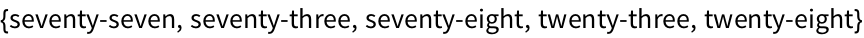Answer & check your solution
Expected output:
 Out[]=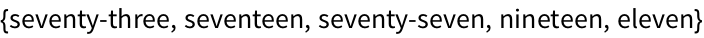Answer & check your solution
Usually list part n or list sub n. The second form (with sub short for subscript) comes from thinking about math and extracting components of vectors.
What happens if one asks for a part of a list that doesnt exist?
One gets a message, and the original computation is returned undone.
Can I just get the first position at which something appears in a list?
• First and Last are equivalent to [] and [[-1]].
• In specifying parts, 1;;-1 is equivalent to All.